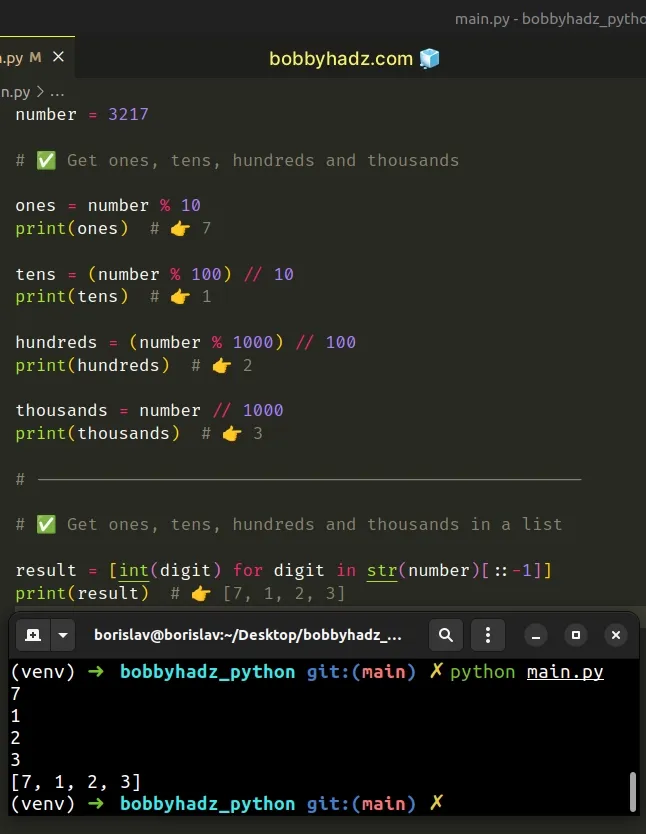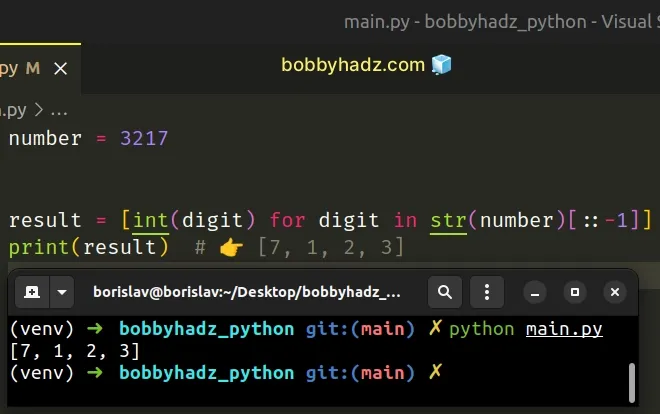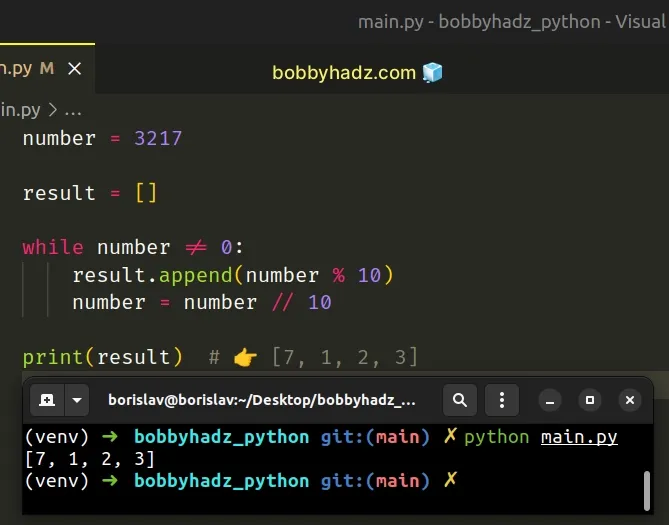# Find the ones, tens, hundreds and thousands digits in PythonLast updated: Feb 21, 2023
3 min## #Find the ones, tens, hundreds and thousands digits in Python

To find the ones, tens, hundreds and thousands:

1. Use the `str()` class to convert the number to a string.
2. Use string slicing to reverse the number.
3. Iterate over the string and return each digit.
main.py
```Copied!```number = 3217

# ✅ Get ones, tens, hundreds and thousands

ones = number % 10
print(ones)  # 👉️ 7

tens = (number % 100) // 10
print(tens)  # 👉️ 1

hundreds = (number % 1000) // 100
print(hundreds)  # 👉️ 2

thousands = number // 1000
print(thousands)  # 👉️ 3

# -----------------------------------------------------

# ✅ Get ones, tens, hundreds and thousands in a list

result = [int(digit) for digit in str(number)[::-1]]
print(result)  # 👉️ [7, 1, 2, 3]
``````The first examples use math to get the ones, tens, hundreds and the thousands digits of a number.

The modulo (%) operator returns the remainder from the division of the first value by the second.

main.py
```Copied!```print(10 % 2)  # 👉️ 0
print(10 % 4)  # 👉️ 2
``````

If the value on the right-hand side is zero, the operator raises a `ZeroDivisionError` exception.

For example, to get the ones, we calculate the remainder of dividing the number by 10.

main.py
```Copied!```number = 3217

ones = number % 10
print(ones)  # 👉️ 7

print(3217 - 321 * 10) # 👉️ 7
``````

We used floor division in the next examples.

Division `/` of integers yields a float, while floor division `//` of integers results in an integer.

The result of using the floor division operator is that of a mathematical division with the `floor()` function applied to the result.

main.py
```Copied!```my_num = 50

print(my_num / 5)  # 👉️ 10.0 (float)
print(my_num // 5)  # 👉️ 10 (int)
``````

For example, to get the thousands, we floor-divide the number by `1000`.

main.py
```Copied!```number = 3217

thousands = number // 1000
print(thousands)  # 👉️ 3
``````

To get the tens, we calculate the remainder of dividing the number by `100` and divide the result by 10.

main.py
```Copied!```number = 3217

print(number % 100) # 👉️ 17

tens = (number % 100) // 10
print(tens)  # 👉️ 1
``````

Similarly, to get the hundreds, we calculate the remainder of dividing the number by `1000` and divide the result by `100`.

main.py
```Copied!```number = 3217

ones = number % 10

print(number % 1000)  # 👉️ 217

hundreds = (number % 1000) // 100
print(hundreds)  # 👉️ 2
``````

## #Find the ones, tens, hundreds and thousands digits using a list comprehension

Alternatively, you can convert the number to a string and use a list comprehension.

main.py
```Copied!```number = 3217

result = [int(digit) for digit in str(number)[::-1]]
print(result)  # 👉️ [7, 1, 2, 3]
``````We used the str() class to convert the number to a string and used string slicing to reverse the string.

The syntax for string slicing is `my_str[start:stop:step]`.

We specified a `step` of `-1` to reverse the string and used a list comprehension to iterate over the reversed string.

List comprehensions are used to perform some operation for every element or select a subset of elements that meet a condition.

The list contains the digits of the ones, tens, hundreds and thousands.

## #Find the ones, tens, hundreds and thousands digits using a while loop

Alternatively, you can use a `while` loop to divide the number by 10 until it gets set to `0`.

main.py
```Copied!```number = 3217

result = []

while number != 0:
result.append(number % 10)
number = number // 10

print(result)  # 👉️ [7, 1, 2, 3]
``````We calculate the digits of the ones, tens, hundreds and thousands and simultaneously floor-divide the number by `10` until it gets set to `0`.